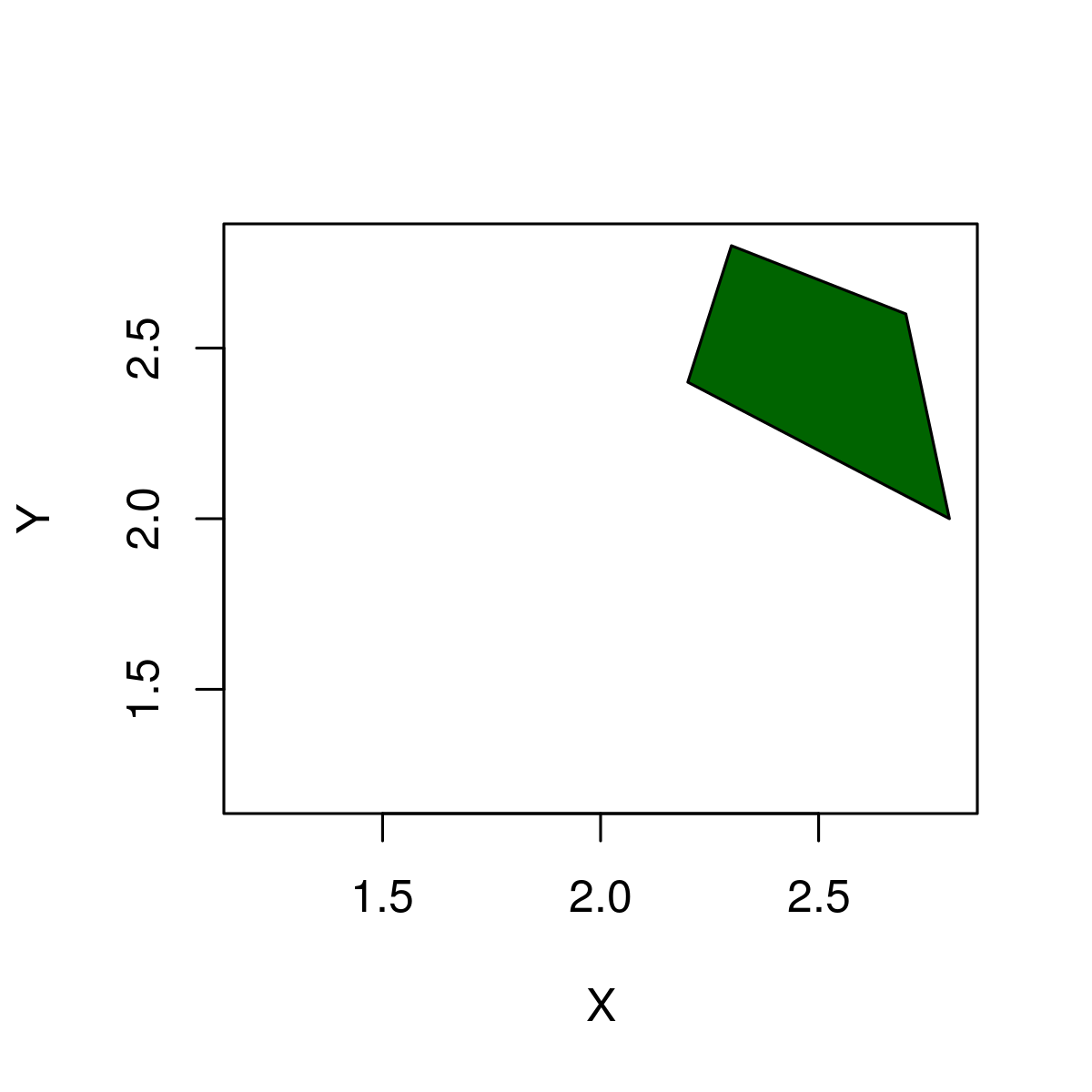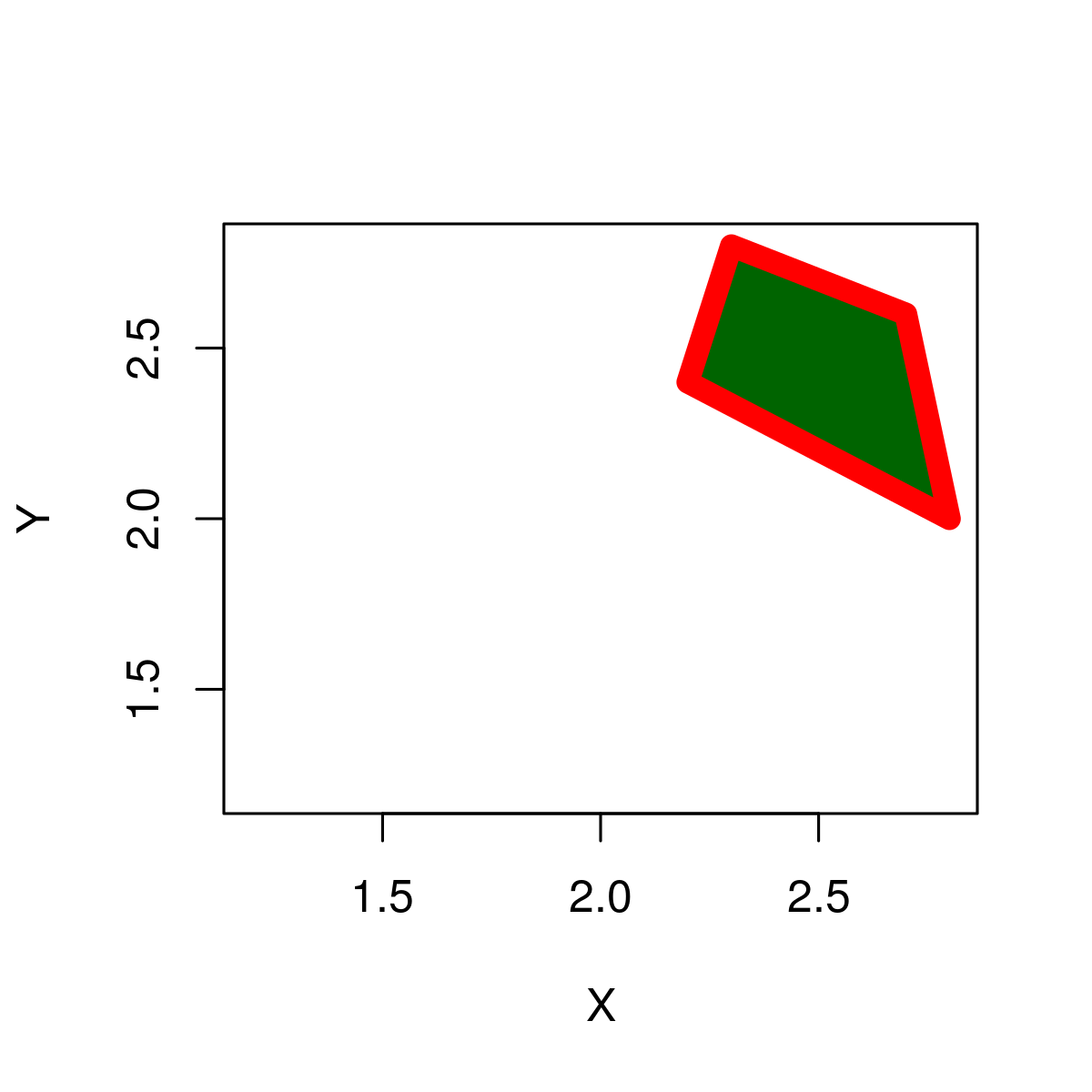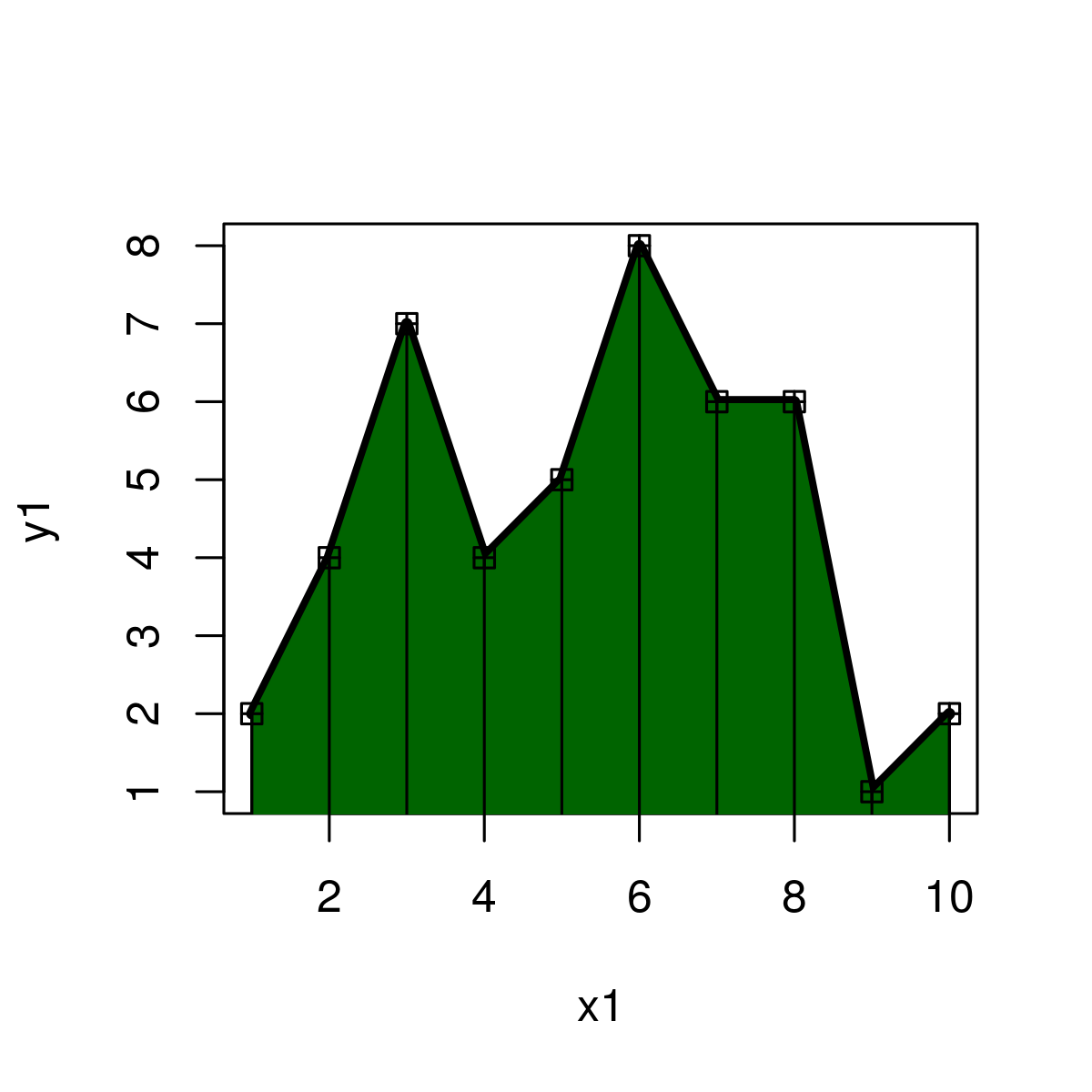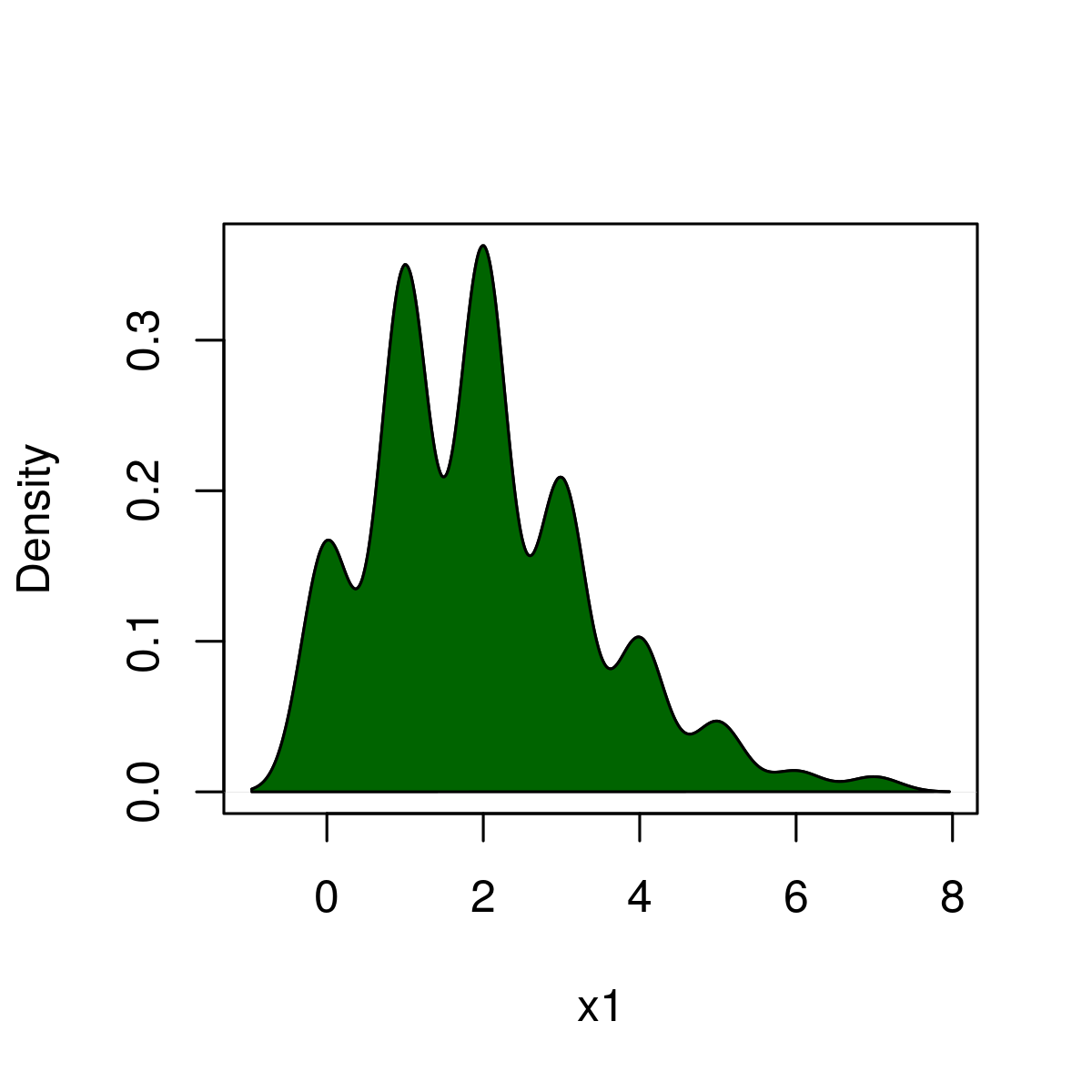# Draw a Polygon between specified points in R Programming – polygon() Function

• Last Updated : 23 Jun, 2020

`polygon()` function in R Language is used to plot a polygon between specified points in an existing plot.

Syntax: polygon(x_coordinates, y_coordinates)

Parameters :
x_coordinates, y_coordinates: x, y coordinates of plot to draw polygon

Returns: a polygon in a given plot

Example 1: Draw a Square Polygon in an R Plot

 `# R program to draw a polygon ` ` `  `# Draw an empty plot ` `plot``(2, 2, col = ``"white"``, xlab = ``"X"``, ylab = ``"Y"``)   ` ` `  `# Draw a polygon ` `polygon``(x = ``c``(2.7, 2.3, 2.2, 2.8),  ``# X-Coordinates of polygon  ` `        ``y = ``c``(2.6, 2.8, 2.4, 2),    ``# Y-Coordinates of polygon ` `        ``col = ``"darkgreen"``)  `

Output:Example 2: Color borders of polygon

 `# R program to draw a polygon ` ` `  `# Draw empty plot ` `plot``(2, 2, col = ``"white"``, xlab = ``"X"``, ylab = ``"Y"``)  ` ` `  `# Draw a polygon ` `polygon``(x = ``c``(2.7, 2.3, 2.2, 2.8),  ``# X-Coordinates of polygon ` `        ``y = ``c``(2.6, 2.8, 2.4, 2),    ``# Y-Coordinates of polygon ` `        ``col = ``"darkgreen"``,          ``# Color of polygon ` `        ``border = ``"red"``,             ``# Color of polygon border ` `        ``lwd = 8)                    ``# Thickness of border `

Output:Here, border specifies the border color and lwd specifies the border thickness.

Example 3: Draw frequency polygon

 `# R program to draw a polygon ` ` `  `# X values for frequency polygon ` `x1 <- 1:10                           ` ` `  `# Y values for frequency polygon ` `y1 <- ``c``(2, 4, 7, 4, 5, 8, 6, 6, 1, 2)   ` ` `  `# Plot frequency polygon ` `plot``(x1, y1,                                        ` `     ``type = ``"l"``,     ``# Set line type to line ` `     ``lwd = 4)         ``# Thickness of line ` `      `  `# X-Y-Coordinates of polygon ` `polygon``(``c``(1, x1, 10), ``c``(0, y1, 0),           ` `        ``col = ``"darkgreen"``)   ``# Color of polygon ` ` `  `# Add squares to frequency polygon ` `points``(x1, y1,                           ` `       ``cex = 1,    ``# Size of squares                              ` `       ``pch = 12)   ` `       ``segments``(x1, 0, x1, y1)   `

Output:Example 4: Draw polygon below density

 `# R program to draw a polygon ` ` `  `# Set seed for reproducibility ` `set.seed``(15000)       ` ` `  `# Sample size ` `N <- 1000              ` ` `  `# Draw random poisson distribution ` `x1 <- ``rpois``(N, 2)                            ` `plot``(``density``(x1),    ``# Draw density plot ` `     ``main = ``""``,      ``# No main title ` `     ``xlab = ``"x1"``)    ``# Set name of x-axis to x2 ` `      `  `# X-Coordinates of polygon ` `polygon``(``c``(``min``(``density``(x1)\$x), ``density``(x1)\$x),            ` `        ``c``(0, ``density``(x1)\$y),     ``# Y-Coordinates of polygon ` `        ``col = ``"darkgreen"``)       ``# Color of polygon `

Output:Here, above example is used to make Probability density function.

My Personal Notes arrow_drop_up
Recommended Articles
Page :